LORENE
Lorene::Eos Class Referenceabstract

Equation of state base class. More...

#include <eos.h>

Inheritance diagram for Lorene::Eos: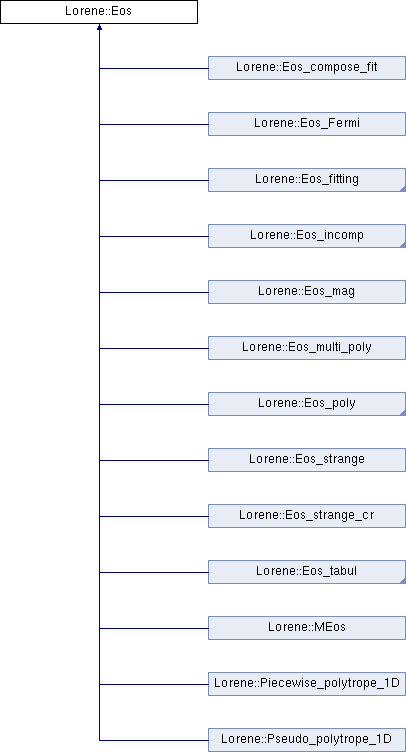## Public Member Functions

virtual ~Eos ()
Destructor. More...

const char * get_name () const
Returns the EOS name. More...

void set_name (const char *name_i)
Sets the EOS name. More...

virtual bool operator== (const Eos &) const =0
Comparison operator (egality) More...

virtual bool operator!= (const Eos &) const =0
Comparison operator (difference) More...

virtual int identify () const =0
Returns a number to identify the sub-classe of Eos the object belongs to. More...

virtual void sauve (FILE *) const
Save in a file. More...

virtual double nbar_ent_p (double ent, const Param *par=0x0) const =0
Computes the baryon density from the log-enthalpy and extra parameters (virtual function implemented in the derived classes). More...

Cmp nbar_ent (const Cmp &ent, int nzet, int l_min=0, Param *par=0x0) const
Computes the baryon density field from the log-enthalpy field and extra parameters. More...

Scalar nbar_ent (const Scalar &ent, int nzet, int l_min=0, Param *par=0x0) const
Computes the baryon density field from the log-enthalpy field and extra parameters. More...

virtual double ener_ent_p (double ent, const Param *par=0x0) const =0
Computes the total energy density from the log-enthalpy and extra parameters (virtual function implemented in the derived classes). More...

Cmp ener_ent (const Cmp &ent, int nzet, int l_min=0, Param *par=0x0) const
Computes the total energy density from the log-enthalpy and extra parameters. More...

Scalar ener_ent (const Scalar &ent, int nzet, int l_min=0, Param *par=0x0) const
Computes the total energy density from the log-enthalpy and extra parameters. More...

virtual double press_ent_p (double ent, const Param *par=0x0) const =0
Computes the pressure from the log-enthalpy and extra parameters (virtual function implemented in the derived classes). More...

Cmp press_ent (const Cmp &ent, int nzet, int l_min=0, Param *par=0x0) const
Computes the pressure from the log-enthalpy and extra parameters. More...

Scalar press_ent (const Scalar &ent, int nzet, int l_min=0, Param *par=0x0) const
Computes the pressure from the log-enthalpy and extra parameters. More...

virtual double der_nbar_ent_p (double ent, const Param *par=0x0) const =0
Computes the logarithmic derivative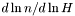from the log-enthalpy and extra parameters (virtual function implemented in the derived classes). More...

Cmp der_nbar_ent (const Cmp &ent, int nzet, int l_min=0, Param *par=0x0) const
Computes the logarithmic derivativefrom the log-enthalpy and extra parameters. More...

Scalar der_nbar_ent (const Scalar &ent, int nzet, int l_min=0, Param *par=0x0) const
Computes the logarithmic derivativefrom the log-enthalpy and extra parameters. More...

virtual double der_ener_ent_p (double ent, const Param *par=0x0) const =0
Computes the logarithmic derivative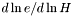from the log-enthalpy with extra parameters (virtual function implemented in the derived classes). More...

Cmp der_ener_ent (const Cmp &ent, int nzet, int l_min=0, Param *par=0x0) const
Computes the logarithmic derivativefrom the log-enthalpy and extra parameters. More...

Scalar der_ener_ent (const Scalar &ent, int nzet, int l_min=0, Param *par=0x0) const
Computes the logarithmic derivativefrom the log-enthalpy and extra parameters. More...

virtual double der_press_ent_p (double ent, const Param *par=0x0) const =0
Computes the logarithmic derivative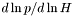from the log-enthalpy and extra parameters (virtual function implemented in the derived classes). More...

Cmp der_press_ent (const Cmp &ent, int nzet, int l_min=0, Param *par=0x0) const
Computes the logarithmic derivativefrom the log-enthalpy and extra parameters. More...

Scalar der_press_ent (const Scalar &ent, int nzet, int l_min=0, Param *par=0x0) const
Computes the logarithmic derivativefrom the log-enthalpy and extra parameters. More...

virtual double csound_square_ent_p (double ent, const Param *par=0x0) const =0
Computes the sound speed squared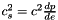from the enthapy with extra parameters (virtual function implemented in the derived classes). More...

Scalar csound_square_ent (const Scalar &ent, int nzet, int l_min=0, Param *par=0x0) const
Computes the sound speed squaredfrom the enthalpy with extra parameters. More...

## Static Public Member Functions

static Eoseos_from_file (FILE *)
Construction of an EOS from a binary file. More...

static Eoseos_from_file (ifstream &)
Construction of an EOS from a formatted file. More...

## Protected Member Functions

Eos ()
Standard constructor. More...

Eos (const char *name_i)
Standard constructor with name. More...

Eos (const Eos &)
Copy constructor. More...

Eos (FILE *)
Constructor from a binary file (created by the function sauve(FILE*) ). More...

Eos (ifstream &)
Constructor from a formatted file. More...

virtual ostream & operator>> (ostream &) const =0
Operator >> More...

void calcule (const Cmp &thermo, int nzet, int l_min, double(Eos::*fait)(double, const Param *) const, Param *par, Cmp &resu) const
General computational method for Cmp 's. More...

void calcule (const Scalar &thermo, int nzet, int l_min, double(Eos::*fait)(double, const Param *) const, Param *par, Scalar &resu) const
General computational method for Scalar 's. More...

## Protected Attributes

char name 
EOS name. More...

## Friends

ostream & operator<< (ostream &, const Eos &)
Display. More...

## Detailed Description

Equation of state base class.

()

Definition at line 206 of file eos.h.

## ◆ Eos() [1/5]

 Lorene::Eos::Eos ( )
protected

Standard constructor.

Definition at line 118 of file eos.C.

References set_name().

## ◆ Eos() [2/5]

 Lorene::Eos::Eos ( const char * name_i )
explicitprotected

Standard constructor with name.

Definition at line 126 of file eos.C.

References set_name().

## ◆ Eos() [3/5]

 Lorene::Eos::Eos ( const Eos & eos_i )
protected

Copy constructor.

Definition at line 134 of file eos.C.

References name, and set_name().

## ◆ Eos() [4/5]

 Lorene::Eos::Eos ( FILE * fich )
protected

Constructor from a binary file (created by the function sauve(FILE*) ).

This constructor is protected because any EOS construction from a binary file must be done via the function Eos::eos_from_file(FILE*) .

Definition at line 142 of file eos.C.

References name.

## ◆ Eos() [5/5]

 Lorene::Eos::Eos ( ifstream & fich )
protected

Constructor from a formatted file.

This constructor is protected because any EOS construction from a formatted file must be done via the function Eos::eos_from_file(ifstream&) .

Definition at line 150 of file eos.C.

References name.

## ◆ ~Eos()

 Lorene::Eos::~Eos ( )
virtual

Destructor.

Definition at line 162 of file eos.C.

## ◆ calcule() [1/2]

 void Lorene::Eos::calcule ( const Cmp & thermo, int nzet, int l_min, double(Eos::*)(double, const Param *) const fait, Param * par, Cmp & resu ) const
protected

General computational method for Cmp 's.

Parameters
 thermo [input] thermodynamical quantity (for instance the enthalpy field)from which the thermodynamical quantity resu is to be computed. nzet [input] number of domains where resu is to be computed. l_min [input] index of the innermost domain is which resu is to be computed [default value: 0]; resu is computed only in domains whose indices are in [l_min,l_min+nzet-1] . In the other domains, it is set to zero. fait [input] pointer on the member function of class Eos which performs the pointwise calculation. par possible extra parameters of the EOS resu [output] result of the computation.

Definition at line 213 of file eos.C.

References Lorene::Cmp::get_etat().

## ◆ calcule() [2/2]

 void Lorene::Eos::calcule ( const Scalar & thermo, int nzet, int l_min, double(Eos::*)(double, const Param *) const fait, Param * par, Scalar & resu ) const
protected

General computational method for Scalar 's.

Parameters
 thermo [input] thermodynamical quantity (for instance the enthalpy field)from which the thermodynamical quantity resu is to be computed. nzet [input] number of domains where resu is to be computed. l_min [input] index of the innermost domain is which resu is to be computed [default value: 0]; resu is computed only in domains whose indices are in [l_min,l_min+nzet-1] . In the other domains, it is set to zero. fait [input] pointer on the member function of class Eos which performs the pointwise calculation. par possible extra parameters of the EOS resu [output] result of the computation.

Definition at line 285 of file eos.C.

References Lorene::Scalar::get_etat().

## ◆ csound_square_ent()

 Scalar Lorene::Eos::csound_square_ent ( const Scalar & ent, int nzet, int l_min = 0, Param * par = 0x0 ) const

Computes the sound speed squaredfrom the enthalpy with extra parameters.

Parameters
 ent [input, unit: c^2] enthalpy nzet number of domains where the derivative dln(e)/dln(H) is to be computed. l_min index of the innermost domain is which the coefficient dln(n)/dln(H) is to be computed [default value: 0]; the derivative dln(e)/dln(H) is computed only in domains whose indices are in [l_min,l_min+nzet-1] . In the other domains, it is set to zero. par possible extra parameters of the EOS
Returns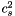[unit: c^2]

Definition at line 499 of file eos.C.

References calcule(), csound_square_ent_p(), and Lorene::Tensor::get_mp().

## ◆ csound_square_ent_p()

 virtual double Lorene::Eos::csound_square_ent_p ( double ent, const Param * par = 0x0 ) const
pure virtual

Computes the sound speed squaredfrom the enthapy with extra parameters (virtual function implemented in the derived classes).

Parameters
 ent [input, unit: c^2] enthalpy par possible extra parameters of the EOS
Returns[unit: c^2]

## ◆ der_ener_ent() [1/2]

 Cmp Lorene::Eos::der_ener_ent ( const Cmp & ent, int nzet, int l_min = 0, Param * par = 0x0 ) const

Computes the logarithmic derivativefrom the log-enthalpy and extra parameters.

Parameters
 ent [input, unit: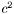] log-enthalpy H defined by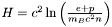, where e is the (total) energy density, p the pressure, n the baryon density, and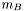the baryon mass nzet number of domains where the derivative dln(e)/dln(H) is to be computed. l_min index of the innermost domain is which the coefficient dln(n)/dln(H) is to be computed [default value: 0]; the derivative dln(e)/dln(H) is computed only in domains whose indices are in [l_min,l_min+nzet-1] . In the other domains, it is set to zero. par possible extra parameters of the EOS
Returns
dln(e)/dln(H)

Definition at line 454 of file eos.C.

References calcule(), der_ener_ent_p(), and Lorene::Cmp::get_mp().

## ◆ der_ener_ent() [2/2]

 Scalar Lorene::Eos::der_ener_ent ( const Scalar & ent, int nzet, int l_min = 0, Param * par = 0x0 ) const

Computes the logarithmic derivativefrom the log-enthalpy and extra parameters.

Parameters
 ent [input, unit:] log-enthalpy H defined by, where e is the (total) energy density, p the pressure, n the baryon density, andthe baryon mass nzet number of domains where the derivative dln(e)/dln(H) is to be computed. l_min index of the innermost domain is which the coefficient dln(n)/dln(H) is to be computed [default value: 0]; the derivative dln(e)/dln(H) is computed only in domains whose indices are in [l_min,l_min+nzet-1] . In the other domains, it is set to zero. par possible extra parameters of the EOS
Returns
dln(e)/dln(H)

Definition at line 464 of file eos.C.

References calcule(), der_ener_ent_p(), and Lorene::Tensor::get_mp().

## ◆ der_ener_ent_p()

 virtual double Lorene::Eos::der_ener_ent_p ( double ent, const Param * par = 0x0 ) const
pure virtual

Computes the logarithmic derivativefrom the log-enthalpy with extra parameters (virtual function implemented in the derived classes).

Parameters
 ent [input, unit:] log-enthalpy H defined by, where e is the (total) energy density, p the pressure, n the baryon density, andthe baryon mass par possible extra parameters of the EOS
Returns
dln(e)/dln(H)

## ◆ der_nbar_ent() [1/2]

 Cmp Lorene::Eos::der_nbar_ent ( const Cmp & ent, int nzet, int l_min = 0, Param * par = 0x0 ) const

Computes the logarithmic derivativefrom the log-enthalpy and extra parameters.

Parameters
 ent [input, unit:] log-enthalpy H defined by, where e is the (total) energy density, p the pressure, n the baryon density, andthe baryon mass nzet number of domains where the derivative dln(n)/dln(H) is to be computed. l_min index of the innermost domain is which the coefficient dln(n)/dln(H) is to be computed [default value: 0]; the derivative dln(n)/dln(H) is computed only in domains whose indices are in [l_min,l_min+nzet-1] . In the other domains, it is set to zero. par possible extra parameters of the EOS
Returns
dln(n)/dln(H)

Definition at line 431 of file eos.C.

References calcule(), der_nbar_ent_p(), and Lorene::Cmp::get_mp().

## ◆ der_nbar_ent() [2/2]

 Scalar Lorene::Eos::der_nbar_ent ( const Scalar & ent, int nzet, int l_min = 0, Param * par = 0x0 ) const

Computes the logarithmic derivativefrom the log-enthalpy and extra parameters.

Parameters
 ent [input, unit:] log-enthalpy H defined by, where e is the (total) energy density, p the pressure, n the baryon density, andthe baryon mass nzet number of domains where the derivative dln(n)/dln(H) is to be computed. l_min index of the innermost domain is which the coefficient dln(n)/dln(H) is to be computed [default value: 0]; the derivative dln(n)/dln(H) is computed only in domains whose indices are in [l_min,l_min+nzet-1] . In the other domains, it is set to zero. par possible extra parameters of the EOS
Returns
dln(n)/dln(H)

Definition at line 441 of file eos.C.

References calcule(), der_nbar_ent_p(), and Lorene::Tensor::get_mp().

## ◆ der_nbar_ent_p()

 virtual double Lorene::Eos::der_nbar_ent_p ( double ent, const Param * par = 0x0 ) const
pure virtual

Computes the logarithmic derivativefrom the log-enthalpy and extra parameters (virtual function implemented in the derived classes).

Parameters
 ent [input, unit:] log-enthalpy H defined by, where e is the (total) energy density, p the pressure, n the baryon density, andthe baryon mass par possible extra parameters of the EOS
Returns
dln(n)/dln(H)

## ◆ der_press_ent() [1/2]

 Cmp Lorene::Eos::der_press_ent ( const Cmp & ent, int nzet, int l_min = 0, Param * par = 0x0 ) const

Computes the logarithmic derivativefrom the log-enthalpy and extra parameters.

Parameters
 ent [input, unit:] log-enthalpy H defined by, where e is the (total) energy density, p the pressure, n the baryon density, andthe baryon mass nzet number of domains where the derivative dln(p)/dln(H) is to be computed. par possible extra parameters of the EOS l_min index of the innermost domain is which the coefficient dln(n)/dln(H) is to be computed [default value: 0]; the derivative dln(p)/dln(H) is computed only in domains whose indices are in [l_min,l_min+nzet-1] . In the other domains, it is set to zero.
Returns
dln(p)/dln(H)

Definition at line 476 of file eos.C.

References calcule(), der_press_ent_p(), and Lorene::Cmp::get_mp().

## ◆ der_press_ent() [2/2]

 Scalar Lorene::Eos::der_press_ent ( const Scalar & ent, int nzet, int l_min = 0, Param * par = 0x0 ) const

Computes the logarithmic derivativefrom the log-enthalpy and extra parameters.

Parameters
 ent [input, unit:] log-enthalpy H defined by, where e is the (total) energy density, p the pressure, n the baryon density, andthe baryon mass nzet number of domains where the derivative dln(p)/dln(H) is to be computed. par possible extra parameters of the EOS l_min index of the innermost domain is which the coefficient dln(n)/dln(H) is to be computed [default value: 0]; the derivative dln(p)/dln(H) is computed only in domains whose indices are in [l_min,l_min+nzet-1] . In the other domains, it is set to zero.
Returns
dln(p)/dln(H)

Definition at line 486 of file eos.C.

References calcule(), der_press_ent_p(), and Lorene::Tensor::get_mp().

## ◆ der_press_ent_p()

 virtual double Lorene::Eos::der_press_ent_p ( double ent, const Param * par = 0x0 ) const
pure virtual

Computes the logarithmic derivativefrom the log-enthalpy and extra parameters (virtual function implemented in the derived classes).

Parameters
 ent [input, unit:] log-enthalpy H defined by, where e is the (total) energy density, p the pressure, n the baryon density, andthe baryon mass par possible extra parameters of the EOS
Returns
dln(p)/dln(H)

## ◆ ener_ent() [1/2]

 Cmp Lorene::Eos::ener_ent ( const Cmp & ent, int nzet, int l_min = 0, Param * par = 0x0 ) const

Computes the total energy density from the log-enthalpy and extra parameters.

Parameters
 ent [input, unit:] log-enthalpy H defined by, where e is the (total) energy density, p the pressure, n the baryon density, andthe baryon mass nzet number of domains where the energy density is to be computed. l_min index of the innermost domain is which the energy density is to be computed [default value: 0]; the energy density is computed only in domains whose indices are in [l_min,l_min+nzet-1] . In the other domains, it is set to zero. par possible extra parameters of the EOS
Returns
energy density [unit: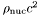], where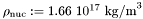Definition at line 387 of file eos.C.

References calcule(), ener_ent_p(), and Lorene::Cmp::get_mp().

## ◆ ener_ent() [2/2]

 Scalar Lorene::Eos::ener_ent ( const Scalar & ent, int nzet, int l_min = 0, Param * par = 0x0 ) const

Computes the total energy density from the log-enthalpy and extra parameters.

Parameters
 ent [input, unit:] log-enthalpy H defined by, where e is the (total) energy density, p the pressure, n the baryon density, andthe baryon mass nzet number of domains where the energy density is to be computed. l_min index of the innermost domain is which the energy density is to be computed [default value: 0]; the energy density is computed only in domains whose indices are in [l_min,l_min+nzet-1] . In the other domains, it is set to zero. par possible extra parameters of the EOS
Returns
energy density [unit:], whereDefinition at line 397 of file eos.C.

References calcule(), ener_ent_p(), and Lorene::Tensor::get_mp().

## ◆ ener_ent_p()

 virtual double Lorene::Eos::ener_ent_p ( double ent, const Param * par = 0x0 ) const
pure virtual

Computes the total energy density from the log-enthalpy and extra parameters (virtual function implemented in the derived classes).

Parameters
 ent [input, unit:] log-enthalpy H defined by, where e is the (total) energy density, p the pressure, n the baryon density, andthe baryon mass par possible extra parameters of the EOS
Returns
energy density e [unit:], where## ◆ eos_from_file() [1/2]

 Eos * Lorene::Eos::eos_from_file ( FILE * fich )
static

Construction of an EOS from a binary file.

The file must have been created by the function sauve(FILE*) .

Definition at line 193 of file eos_from_file.C.

## ◆ eos_from_file() [2/2]

 Eos * Lorene::Eos::eos_from_file ( ifstream & fich )
static

Construction of an EOS from a formatted file.

The fist line of the file must start by the EOS number, according to the following conventions:

• 1 = relativistic polytropic EOS (class Eos_poly ).
• 2 = Newtonian polytropic EOS (class Eos_poly_newt ).
• 3 = Relativistic incompressible EOS (class Eos_incomp ).
• 4 = Newtonian incompressible EOS (class Eos_incomp_newt ).
• 5 = Strange matter (MIT Bag model)
• 6 = Strange matter (MIT Bag model) with crust
• 10 = SLy4 (Douchin & Haensel 2001)
- 11 = FPS (Friedman-Pandharipande + Skyrme)
• 12 = BPAL12 (Bombaci et al. 1995)
• 13 = AkmalPR (Akmal, Pandharipande & Ravenhall 1998)
• 14 = BBB2 (Baldo, Bombaci & Burgio 1997)
• 15 = BalbN1H1 (Balberg 2000)
• 16 = GlendNH3 (Glendenning 1985, case 3)
• 17 = Tabulated EOS in CompOSE format
• 18 = magnetized (tabulated) equation of state
• 19 = relativistic ideal Fermi gas at zero temperature (class Eos_Fermi)
• 20 = Tabulated EOS in CompOSE format corrected for thermo. consistency
• 100 = Multi-domain EOS (class MEos )
• 110 = Multi-polytropic EOS (class Eos_multi_poly )
• 120 = Fitted SLy4 (Shibata 2004)
• 121 = Fitted FPS (Shibata 2004)
• 122 = Fitted AkmalPR (Taniguchi 2005)

The second line in the file should contain a name given by the user to the EOS. The following lines should contain the EOS parameters (one parameter per line), in the same order than in the class declaration.

Definition at line 345 of file eos_from_file.C.

## ◆ get_name()

 const char * Lorene::Eos::get_name ( ) const

Returns the EOS name.

Definition at line 179 of file eos.C.

References name.

## ◆ identify()

 virtual int Lorene::Eos::identify ( ) const
pure virtual

Returns a number to identify the sub-classe of Eos the object belongs to.

## ◆ nbar_ent() [1/2]

 Cmp Lorene::Eos::nbar_ent ( const Cmp & ent, int nzet, int l_min = 0, Param * par = 0x0 ) const

Computes the baryon density field from the log-enthalpy field and extra parameters.

Parameters
 ent [input, unit:] log-enthalpy H defined by, where e is the (total) energy density, p the pressure, n the baryon density, andthe baryon mass nzet number of domains where the baryon density is to be computed. l_min index of the innermost domain is which the baryon density is to be computed [default value: 0]; the baryon density is computed only in domains whose indices are in [l_min,l_min+nzet-1] . In the other domains, it is set to zero. par possible extra parameters of the EOS
Returns
baryon density [unit: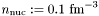]

Definition at line 362 of file eos.C.

References calcule(), Lorene::Cmp::get_mp(), and nbar_ent_p().

## ◆ nbar_ent() [2/2]

 Scalar Lorene::Eos::nbar_ent ( const Scalar & ent, int nzet, int l_min = 0, Param * par = 0x0 ) const

Computes the baryon density field from the log-enthalpy field and extra parameters.

Parameters
 ent [input, unit:] log-enthalpy H defined by, where e is the (total) energy density, p the pressure, n the baryon density, andthe baryon mass nzet number of domains where the baryon density is to be computed. l_min index of the innermost domain is which the baryon density is to be computed [default value: 0]; the baryon density is computed only in domains whose indices are in [l_min,l_min+nzet-1] . In the other domains, it is set to zero. par possible extra parameters of the EOS
Returns
baryon density [unit:]

Definition at line 372 of file eos.C.

References calcule(), Lorene::Tensor::get_mp(), and nbar_ent_p().

## ◆ nbar_ent_p()

 virtual double Lorene::Eos::nbar_ent_p ( double ent, const Param * par = 0x0 ) const
pure virtual

Computes the baryon density from the log-enthalpy and extra parameters (virtual function implemented in the derived classes).

Parameters
 ent [input, unit:] log-enthalpy H defined by, where e is the (total) energy density, p the pressure, n the baryon density, andthe baryon mass par possible extra parameters of the EOS
Returns
baryon density [unit:]

## ◆ operator!=()

 virtual bool Lorene::Eos::operator!= ( const Eos & ) const
pure virtual

## ◆ operator==()

 virtual bool Lorene::Eos::operator== ( const Eos & ) const
pure virtual

## ◆ operator>>()

 virtual ostream& Lorene::Eos::operator>> ( ostream & ) const
protectedpure virtual

## ◆ press_ent() [1/2]

 Cmp Lorene::Eos::press_ent ( const Cmp & ent, int nzet, int l_min = 0, Param * par = 0x0 ) const

Computes the pressure from the log-enthalpy and extra parameters.

Parameters
 ent [input, unit:] log-enthalpy H defined by, where e is the (total) energy density, p the pressure, n the baryon density, andthe baryon mass nzet number of domains where the pressure is to be computed. l_min index of the innermost domain is which the pressure is to be computed [default value: 0]; the pressure is computed only in domains whose indices are in [l_min,l_min+nzet-1] . In the other domains, it is set to zero. par possible extra parameters of the EOS
Returns
pressure [unit:], whereDefinition at line 409 of file eos.C.

References calcule(), Lorene::Cmp::get_mp(), and press_ent_p().

## ◆ press_ent() [2/2]

 Scalar Lorene::Eos::press_ent ( const Scalar & ent, int nzet, int l_min = 0, Param * par = 0x0 ) const

Computes the pressure from the log-enthalpy and extra parameters.

Parameters
 ent [input, unit:] log-enthalpy H defined by, where e is the (total) energy density, p the pressure, n the baryon density, andthe baryon mass nzet number of domains where the pressure is to be computed. l_min index of the innermost domain is which the pressure is to be computed [default value: 0]; the pressure is computed only in domains whose indices are in [l_min,l_min+nzet-1] . In the other domains, it is set to zero. par possible extra parameters of the EOS
Returns
pressure [unit:], whereDefinition at line 419 of file eos.C.

References calcule(), Lorene::Tensor::get_mp(), and press_ent_p().

## ◆ press_ent_p()

 virtual double Lorene::Eos::press_ent_p ( double ent, const Param * par = 0x0 ) const
pure virtual

Computes the pressure from the log-enthalpy and extra parameters (virtual function implemented in the derived classes).

Parameters
 ent [input, unit:] log-enthalpy H defined by, where e is the (total) energy density, p the pressure, n the baryon density, andthe baryon mass par possible extra parameters of the EOS
Returns
pressure p [unit:], where## ◆ sauve()

 void Lorene::Eos::sauve ( FILE * fich ) const
virtual

Save in a file.

Definition at line 189 of file eos.C.

References Lorene::fwrite_be(), identify(), and name.

## ◆ set_name()

 void Lorene::Eos::set_name ( const char * name_i )

Sets the EOS name.

Definition at line 173 of file eos.C.

References name.

## ◆ operator<<

 ostream& operator<< ( ostream & ost, const Eos & eqetat )
friend

Display.

Definition at line 201 of file eos.C.

## ◆ name

 char Lorene::Eos::name
protected

EOS name.

Definition at line 212 of file eos.h.

The documentation for this class was generated from the following files: